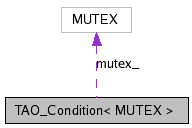# TAO_Condition< MUTEX > Class Template Reference

Same as to the ACE_Condition variable wrapper. More...

`#include <Condition.h>`

Collaboration diagram for TAO_Condition< MUTEX >:[legend]
List of all members.

## Public Types

typedef MUTEX LOCK
Useful typedef.

## Public Member Functions

TAO_Condition (MUTEX &m)
Initialize the condition variable.
TAO_Condition (void)
~TAO_Condition (void)
Implicitly destroy the condition variable.
int wait (const ACE_Time_Value *abstime)
int wait (void)
Block on condition.
int wait (MUTEX &mutex, const ACE_Time_Value *abstime=0)
int signal (void)
int remove (void)
Explicitly destroy the condition variable.
MUTEX * mutex (void)
Returns a reference to the underlying mutex_;.

## Private Member Functions

void operator= (const TAO_Condition< MUTEX > &)
TAO_Condition (const TAO_Condition< MUTEX > &)

## Private Attributes

MUTEX * mutex_
Reference to mutex lock.
int delete_lock_
A flag to indicate whether the lock needs to be deleted.
TAO_SYNCH_CONDITION * cond_
Condition variable.

## Detailed Description

### template<class MUTEX> class TAO_Condition< MUTEX >

Same as to the ACE_Condition variable wrapper.

This class differs from ACE_Condition in that it uses a TAO_SYNCH_CONDITION instead of ACE_cond_t under the hood to provide blocking.

## Member Typedef Documentation

template<class MUTEX>
 typedef MUTEX TAO_Condition< MUTEX >::LOCK

Useful typedef.

## Constructor & Destructor Documentation

template<class MUTEX>
 TAO_BEGIN_VERSIONED_NAMESPACE_DECL TAO_Condition< MUTEX >::TAO_Condition ( MUTEX & m ) ` [inline]`

Initialize the condition variable.

template<class MUTEX>
 TAO_Condition< MUTEX >::TAO_Condition ( void ) ` [inline]`

A default constructor. Since no lock is provided by the user, one will be created internally.

template<class MUTEX>
 TAO_Condition< MUTEX >::~TAO_Condition ( void ) ` [inline]`

Implicitly destroy the condition variable.

template<class MUTEX>
 TAO_Condition< MUTEX >::TAO_Condition ( const TAO_Condition< MUTEX > & ) ` [private]`

## Member Function Documentation

template<class MUTEX>
 ACE_INLINE int TAO_Condition< MUTEX >::wait ( const ACE_Time_Value * abstime ) ` [inline]`

Block on condition, or until absolute time-of-day has passed. If abstime == 0 use "blocking" <wait> semantics. Else, if <abstime> != 0 and the call times out before the condition is signaled <wait> returns -1 and sets errno to ETIME.

template<class MUTEX>
 TAO_BEGIN_VERSIONED_NAMESPACE_DECL ACE_INLINE int TAO_Condition< MUTEX >::wait ( void ) ` [inline]`

Block on condition.

template<class MUTEX>
 ACE_INLINE int TAO_Condition< MUTEX >::wait ( MUTEX & mutex, const ACE_Time_Value * abstime = `0` ) ` [inline]`

Block on condition or until absolute time-of-day has passed. If abstime == 0 use "blocking" wait() semantics on the <mutex> passed as a parameter (this is useful if you need to store the <Condition> in shared memory). Else, if <abstime> != 0 and the call times out before the condition is signaled <wait> returns -1 and sets errno to ETIME.

template<class MUTEX>
 ACE_INLINE int TAO_Condition< MUTEX >::signal ( void ) ` [inline]`

template<class MUTEX>
 ACE_INLINE int TAO_Condition< MUTEX >::broadcast ( void ) ` [inline]`

template<class MUTEX>
 ACE_INLINE int TAO_Condition< MUTEX >::remove ( void ) ` [inline]`

Explicitly destroy the condition variable.

template<class MUTEX>
 ACE_INLINE MUTEX * TAO_Condition< MUTEX >::mutex ( void ) ` [inline]`

Returns a reference to the underlying mutex_;.

template<class MUTEX>
 void TAO_Condition< MUTEX >::operator= ( const TAO_Condition< MUTEX > & ) ` [private]`

## Member Data Documentation

template<class MUTEX>
 MUTEX* TAO_Condition< MUTEX >::mutex_` [private]`

Reference to mutex lock.

template<class MUTEX>
 int TAO_Condition< MUTEX >::delete_lock_` [private]`

A flag to indicate whether the lock needs to be deleted.

template<class MUTEX>
 TAO_SYNCH_CONDITION* TAO_Condition< MUTEX >::cond_` [private]`

Condition variable.

The documentation for this class was generated from the following files:
Generated on Mon Jun 25 16:39:23 2007 for TAO by1.5.2-2